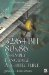Packed Integer MultiplicationPacked integer multiplication is one of the mathematical equations that you will tend to use in your SIMD application either as fixed-point or just parallel integer processing. This works out nicely when it is necessary to increase the magnitude of a series of integers. The problem here comes up because fixed-point multiplication is not like floating-point multiplication. In floating-point, there is a precision loss with each calculation since a numerical value is stored in an exponential form. With fixed-point, there is no precision loss, which is great but leads to another problem. When two integers are used in a summation, the most significant bits are carried into an additional (n+1) bit. With a multiplication of two integers, the resulting storage required is (n+n=2n) bits. This poses a problem of how to deal with the resulting solution. Since the data size increases , there are multiple solutions to contain the result of the calculation.

HI(int16—int16)

HI(uint16—uint16)

LO(int16—int16)

pmulhw

pmulhuw

pmullw

• Store upper bits.

• Store lower bits.

• Store upper/lower bits into two vectors.

• Store even n bit elements into 2n bit elements.

• Store odd n bit elements into 2n bit elements.

PMULLW N—16-Bit Parallel Multiplication (Lower)

PMULLW destination , sourceMnemonic

P

PII

K6

3D!

3Mx+

SSE

SSE2

A64

SSE3

E64T

PMULLW32/64-Bit 80x86 Assembly Language Architecture
ISBN: 1598220020
EAN: 2147483647
Year: 2003
Pages: 191

Similar book on Amazon